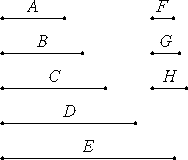# Proposition 6

If there are as many numbers as we please in continued proportion, and the first does not measure the second, then neither does any other measure any [later] other.

Let there be as many numbers as we please, A, B, C, D, and E, in continued proportion, and let A not measure B.

I say that neither does any other measure any [later] other.

Now it is manifest that A, B, C, D, and E do not measure one another in order, for A does not even measure B.

I say, then, that neither does any other measure any [later] other.VII.33

If possible, let A measure C. And, however many A, B, and C are, take as many numbers F, G, and H, the least of those which have the same ratio with A, B, and C.

VII.14

Now, since F, G, and H are in the same ratio with A, B, and C, and the multitude of the numbers A, B, and C equals the multitude of the numbers F, G, and H, therefore, ex aequali A is to C as F is to H.

VII.Def.20

And since A is to B as F is to G, while A does not measure B, therefore neither does F measure G. Therefore F is not a unit, for the unit measures any number.

VIII.3

Now F and H are relatively prime. And F is to H as A is to C, therefore neither does A measure C.

Similarly we can prove that neither does any other measure any other.

Therefore, if there are as many numbers as we please in continued proportion, and the first does not measure the second, then neither does any other measure any other.

Q.E.D.

## Guide

The proposition as stated isn’t quite correct. For example, the numbers 24, 12, 6 and 3 are in continued proportion, and 24 does not divide 12, but each of the others does divide others, for instance, 3 divides 6. But none of the others divide others later in the sequence. Thus, the statement of the theorem needs the bracketed word later.

#### Outline of the proof

Consider a sequence of numbers in continued proportion where the first number does not divide the second. Since any number in that sequence has to the next number in the sequence the same ratio as the first has to the second, therefore no number divides the next.

Suppose that some number in the sequence divides a later number. We may call that the former number a since it divides the next number in the sequence, and call the number it divides c. Take the continued proportion a, b, ..., c and, using VII.33, reduce it a continued proportion f, g, ..., h in lowest terms. Since that’s in lowest terms, f and h are relatively prime. Since a : b = f : g, and a does not divide b, therefore f does not divide g. Since f does not divide g, in particular f does not equal 1, but f and h are relatively prime by VIII.3, therefore f does not divide h. Finally, since a : c = f : h, therefore a does not divide c either.

#### Use of this proposition

This proposition is used as a lemma for the following proposition.